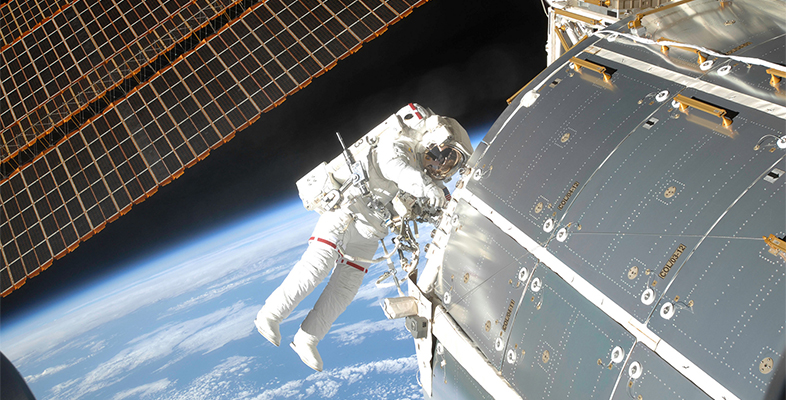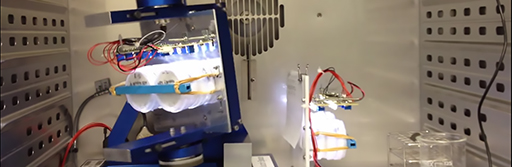Microgravity: living on the International Space Station

Start this free course now. Just create an account and sign in. Enrol and complete the course for a free statement of participation or digital badge if available.

Free course

# 4 Random positioning machines

Random positioning machines or RPMs (Figure 4) are used for research into:

• cell biology
• microbiology
• regenerative medicine
• tissue engineering and stem cells
• experimenting with bacteria in a microgravity environment.Figure 4 A random positioning machine (RPM).

An RPM simulates microgravity by rotating with random speeds in all directions. This makes the sample experience gravity from every direction. Over a period of time, the average acceleration due to gravity is zero. The RPM can also provide a different value of gravity where organisms or cells can change.

An RPM can simulate the numerical values of gravity different from Earth’s gravity g (9.81 m/s2). In the case of Mars, this is equivalent to 0.38 g. In the case of the Moon, this is equivalent to 0.18 g.

Now, using these values, complete Activity 5.

## Activity 5 RPM and values of gravity

Timing: Allow approximately 15 minutes

Answer the following questions by choosing one correct option.

1. What is the numerical value of the acceleration due to gravity on the Moon?

a.

3.54 m/s2

b.

2.77 m/s2

c.

0.77 m/s2

d.

1.77 m/s2

e.

177 m/s2

2. What is the numerical value of the acceleration due to gravity on Mars?

a.

3.73 m/s2

b.

7.46 m/s2

c.

37.3 m/s2

d.

373 m/s2

e.

0.373 m/s2

3. In Week 1, you calculated the weight of an object with the same mass on different planets. Which unit for gravitational field strength did you use?

a.

N

b.

kg

c.

N/kg

d.

m/s

e.

m

You will now look at the survivability of microbes in extreme physical conditions on Earth and elsewhere in the Universe.

MG_1